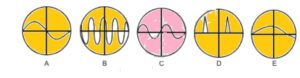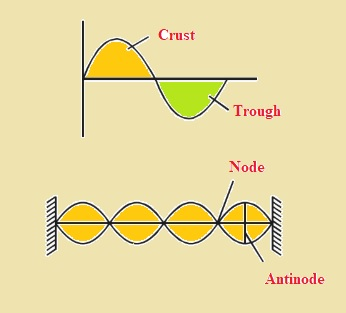Hello, studnets welcome to new post. Here we will discuss Fsc 1st Year Physics Chapter 8 Solved Exercise. Here we will learn chapter 8 exercise questions in simple way that will be helpful for you solve your papers. So let get started

## Fsc 1st Year Physics Chapter 8 Solved Exercise

Q1: Solution

• The similarity between transverse waves and longitudinal waves are listed here
• These are mechanical waves
• These two waves transfer energy from one point to other without the transfer of matter.
• These waves create disturbance in a medium where they created
• Equation V=fλ applied for both waves

Q2: Solution• Trace B tells about the loudest sound since loudness depends on the vibration of sound
• Trace B explains the higest value of frequency since the number of waves per second are highest

Q3: Solution

• There is no production of stationary waves if two waves are traveling in the same direction.
• For the creation of stationary waves, there must be an opposite direction of waves.

Q4:Solution

• That will be stationary waves since some points in stationary waves are rest. Zero value of displacement known as a node

Q5:Solution• The highest point of transverse waves from the mean point is called the crust
• The lowest point of transverse waves from the mean point is called trough
• Point, where stationary waves has zero displacements, is called node.
• The point where displacement of transverse waves is high called antinode.

Q6: Solution

• The equation for speed of sound is
• V=√E/Ρ
• According to this equation speed of sound is directly proportional to the square root of modules of elasticity. Value of elasticity and density ia high for solids than gases. Therefore elasticity effect is larger than density so sound travels faster in solids than in gases.

Q7:Solution

• By varying the length of air column, numerous notes can be created.
• To get a certain level of note standard instrument taken and sounded with the instrument that has to tune. Beats created in one second are recorded.
• The frequency of the instrument to be tuned s adjusted so it not give any beat with a standard instrument
• So musical instruments are tuned to a certain frequency through the phenomenon of beats.

Q8:Solution

• The number of beats created in one second is equal to difference between values of 2 note frequencies
• The number of beats in one seconds = f1-f2 iii option is correct

Q9:Solution

• We know that speed of sound is larger in solids than in gases. So time difference through ground is is faster than speed through gases.

Q10:Solution

• The equation for sound of speed in air is
• V=√rp/ρ
• Here we can observe that speed is inverse to √ρ, Which indicates that if the density is larger speed will be less. The density of smaller air is less so speed is high

Q11:Solution

• If observer and sound waves are moving in the same direction and having same value of velocity in result relative velocity is 0. So frequency of sound not changes.

I have explained Fsc 1st Year Physics Chapter 8 Solved Exercise. If you have any further query ask in comments thanks for reading have a nice day.